Module 8 - Derivative of a Function

Introduction | Lesson 1 | Lesson 2 | Lesson 3 | Self-Test

Lesson 8.2: Local Linearity

In Lesson 8.1 you found the equation of the line tangent to f(x) = 2xx2 at (0.5, 0.75). In this lesson you will explore the appearance of the graphs of y = f(x) and its tangent line as you zoom in around the point (0.5, 0.75).

First reset the factory defaults. Click here to see the keystroke sequence to do this.

Zooming In

• Display the graphs of y1 = f(x) and y2 = (x–0.5) + 0.75 in a [-1, 3] x [-1, 2] window with xscl and yscl = 1
These are the same functions that were drawn in Lesson 8.1, so if the functions are still there you may just set the window coordinates.
• Select "2:ZoomIn" from the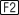Zoom menu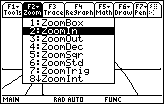The TI-89 prompts you for the zoom center.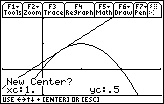• Move the cursor as close as you can to (0.5, 0.75) by using the cursor movement keys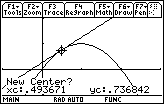• Zoom in on the point you have selected by pressing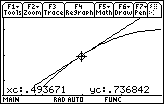The graphs are redrawn in a smaller window whose center is approximately (0.5, 0.75).

Zooming in Again

The local linearity of the function can be better illustrated by zooming in repeatedly.

• Select "2:ZoomIn" from the Zoom menu
• Move the cursor as close as you can to (0.5, 0.75)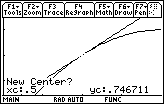• Zoom in by pressing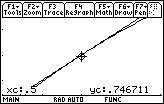• Repeat the zoom in process once more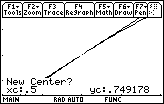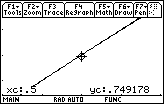Select a center close to (0.5, 0.75) Zoom in on the selected point

In the last window the graphs of the function and the tangent line appear to coincide. That is, the curve appears to be linear on a small scale.

Local Linearity and Point Differentiability

If a curve looks linear around a point after zooming in repeatedly, it is said to be locally linear near that point. If a curve is locally linear near a point, the curve is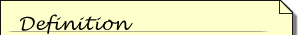A function is differentiable at a point if its derivative exits there.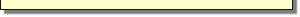differentiable at the point.

Determining Differentiability Graphically

One of the following functions is differentiable at x = 0 and the other is not: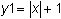and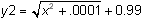Displaying the graphs of the functions illustrates which one is differentiable.

• Set y1 = abs(x)+1
The absolute value command can be found in the catalog or typed in one letter at a time.
• Set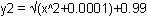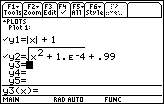• Display the graphs in a Decimal window by selecting 4:ZoomDec from theZoom menu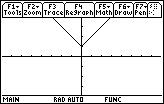The graphs appear to coincide in this viewing window. You may want to use Trace to convince yourself that both graphs are present.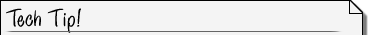Identifying Graphs While using Trace you may toggle between the graphs by using the cursor movement keys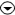and. A small number in the upper right corner of the Graph screen denotes the selected graph.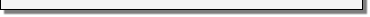Setting the Zoom Factors

Zoom in around the point (0,1) to see which graph is locally linear there. You may want a higher level of magnification to make the process faster. This can be accomplished by changing the Zoom factors.

• Display the Zoom menu by pressing• Scroll down until "C:SetFactors" is highlighted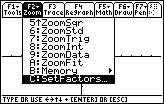• Open the Zoom Factors setup window by pressing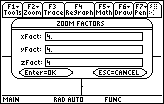• Change xFACT and yFACT in the third row from 4 to 10.
We will leave xFACT and yFACT set to 4 for the remainder of the lesson.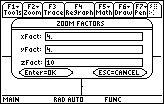• If you want to change xFact and yFact, then save the new factors by pressing• Exit the set up window and return to the graph by pressingZooming In Using the New Factors

• Repeatedly zoom in on the point (0,1) by using the procedure described earlier

At first the graphs will appear to be identical, but as you continue to zoom in, you should see a difference.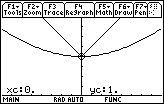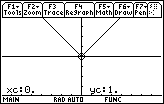After zooming in 5 times After zooming in 8 times

8.2.1 Based on the graphs above, which function is differentiable at x = 0 and which is not? Click here for the answer.

< Back | Next >